### Home > A2C > Chapter 4 > Lesson 4.2.1 > Problem4-72

4-72.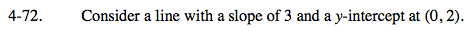Use the eTool below to graph the line.
Click the link at right for the full version of the eTool: A2C 4-72 HW eTool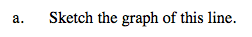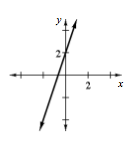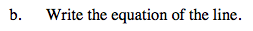Try using a slope-intercept equation.

y = mx + b

Use the slope and y-intercept to make the equation.

y = 3x + 2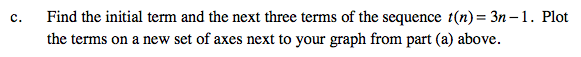Substitute 1, 2, 3, and 4 for n to find the terms.

t(1) = 3 –1 t(2) = 6 –1 t(3) = 9 –1 t(4) = 12 – 1

Graph the results.

t(1) = 2 t(2) = 5 t(3) = 8 t(4) = 11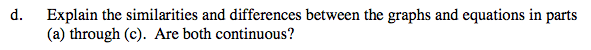Notice that both graphs have the same slope.

The graphs are parallel, but they have different intercepts. The first is continuous and the second is discrete.